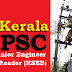## Recent Posts

1. On which law electrical energy is generated

(a) Flemings Law
(b) Ohm's Law
(c) Faraday's Law of Electromagnetic induction
(d) Lenz's Law

2. The direction of dynamically induced emf can be identified by using

(a) Lenz's Law
(b) Fleming's right hand rule
(c) Fleming's left hand rule
(d) Faraday's law of electromagnetic induction

3. Commutator segments of D.C. machine are made of

(a) Soft Iron
(b) Polished silver
(c) Hard drawn copper
(d) Graphic

4. The function of yoke in a DC machine is

(a) Act as a protective cover
(b) The path for completing magnetic flux
(c) both (a) and (b)
(d) None of these

5. The function of commutator in DC generator is

(a) To give supply to generator
(b) To convert induced emf to unidirectional flow
(c) To avoid Sparking
(d) To improve efficiency

6. Large, dia and smaller axial length of generator is suitable for

(a) Slow speed revolution
(b) High speed revolution
(c) Synchronous speed
(d) Sub Synchronous speed

7. Induced emf in a DC generator

(a) Increase as flux increases
(b) Increase as speed of the prime mover reduces
(c) Increases as field increases
(d) Remain same irrespective of flux and speed

8. Why the armature is laminated?

(a) To reduce heat
(b) To reduce eddy current
(c) To reduce magnetic flux
(d) To increase magnetic flux

9. The starting winding of a single phase motor is placed in the

(a) Rotor
(b) Stator
(c) armature
(d) field

10. In a synchronous motor damper windings are provided in order to

(a) Stabilize rotor motion
(b) Suppress rotor oscillation
(c) develop necessary starting torque
(d) both (b) and (c)

11. A universal motor is one which

(a) is available universally
(b) Run at dangerously high speed on on-load
(c) can be operated either AC or DC
(d) can be marketed internationally

12. Which loss of DC generator varies with load?

(a) Eddy current loss
(b) Hysteresis loss
(c) Copper loss
(d) windage loss

13. Interpoles in DC machines are used for

(a) Increasing voltage
(b) increasing current
(c) Balancing induced emf
(d) Improving commutation

14. OCC is a graph which gives the relation between

(a) E0and IF
(b) V and IL
(c) V and la
(d) Egand la

15. A six pole generator has six parallel path. The generator is wound in

(a) Lap winding
(b) Wave winding
(c) Concentric winding
(d) bucket winding

16. When a motor is working in synchronous speed its percentage slip is

(a) Zero
(b) 100%
(c) Infinity
(d) none of these

17. Alternator are related

(a) kW
(b) kVA
(c) kVAr
(d) HP

18. The relation between frequency, speed and number of poles of an alternator

(a) f=120/PN
(b) f=N/120
(c) N=120P/f
(d) f=PN/120

19. The motor used for portable electric drilling machine

(a) Repulsion motor
(c) Universal motor
(d) capacitor start induction motor

20. Induction motor run at

(a) Synchronous speed
(b) Above Synchronous speed
(c) Below Synchronous speed
(d) Very low speed

21. The transformers are working on the principle of

(a) Mutual induction
(b) Conversion of Energy
(c) Self-induction
(d) Eddy current

22. No-load test on a transformer is carried out to determine

(a) Copper loss
(b) magnetizing current
(c) magnetizing current and no load loss
(d) Efficiency of the transformer

23. A transformer varies the output

(a) Current
(b) Frequency
(c) Power
(d) Energy

24. The motor used for traction purpose

(a) Shunt motor
(b) Series motor
(c) Induction motor
(d) Universal motor

25. The value of transformation ratio of step-up transformer will be

(a) Less than 1
(b) unity
(c) more than
(d) none of these

26. The main flux in a generator distorts due to

(b) Current in the commutator
(c) Current in the armature conductor
(d) Current in the field coil

27. In a transformer the voltage induced in the secondary winding

(a) lead the flux by 90 degree
(b) lag the flu by 90 degree
(c) lead the flux by less than 45 degree
(d) Lags the flux by less than 5 degree

28. Auto transformers are used to

(a) Start DC motor
(b) Boost voltage level
(c) Isolate power ciruit
(d) HV transmission

29. Dummy coil used in the armature winding to

(a) Neutralize armature reaction
(b) Provide mechanical balancing
(c) make self-starting
(d) make sparkles communication

30. High frequency transformer core are generally made from

(a) Ainico
(b) Cobalt
(c) Ferrites
(d) Silicon steel

Electrical Engineering for AE-Electrical, SSC Junior Engineer, Meter Reader (KSEB)Reviewed by Unknown on 9:11 PM Rating: 5